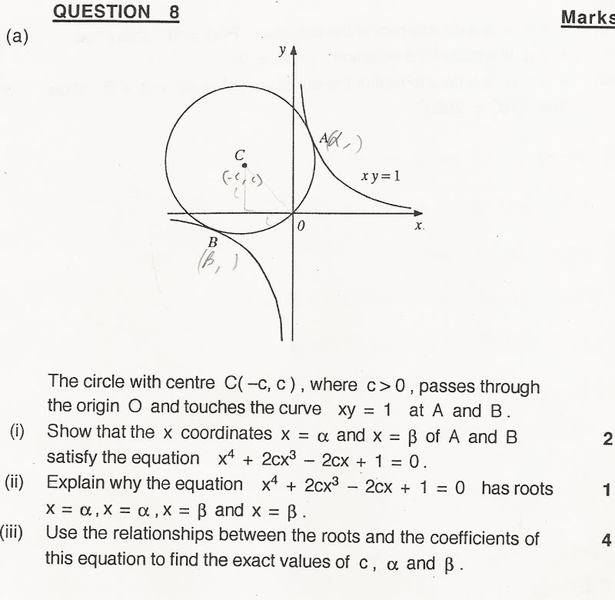# Relationship between roots and coefficients

## Homework Statement## Homework Equations

Sum of roots taken one at a time is -b/a
Sum of roots taken two at a time is c/a
three at a time is -d/a
four at a time is e/a

## The Attempt at a Solution

I did part one by solving the two equations simultaneously.
For part two, I said that it has those roots because that is where the two curves touch
I'm stuck on part three - tried to solve it by applying the above equations and eliminating $\gamma$ and $\delta$ since they are equal to $\alpha$ and $\beta$ respectively but this did not work.
Help would be greatly appreciated :)

SammyS
Staff Emeritus
Homework Helper
Gold Member

## Homework Equations

Sum of roots taken one at a time is -b/a
Sum of roots taken two at a time is c/a
three at a time is -d/a
four at a time is e/a

## The Attempt at a Solution

I did part one by solving the two equations simultaneously.
For part two, I said that it has those roots because that is where the two curves touch
I'm stuck on part three - tried to solve it by applying the above equations and eliminating $\gamma$ and $\delta$ since they are equal to $\alpha$ and $\beta$ respectively but this did not work.
Help would be greatly appreciated :)

For (i). Solving the equations simultaneously only means that the points satisfying that equation are on both the circle and one hyperbola. It doesn't mean that such points occur where the curves are tangent to each other.

Since this is in the pre-calculus section, I ask, do you know how to show that the points of intersection are points of tangency ?

The question says that the curves touch at the points A and B so I assumed they were tangential to each other at those points. Not sure how I would prove it otherwise seeing as I only have an x value for the points.

SammyS
Staff Emeritus